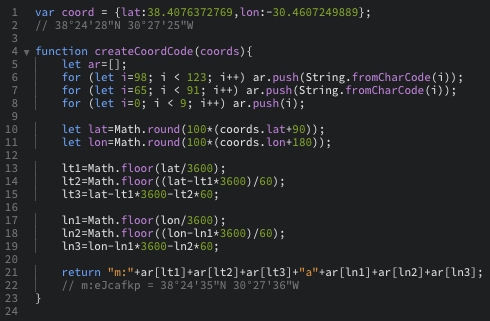# How to create marker on URL

• https://www.windy.com/?gfs,38.284,-37.430,8,i:pressure,m:eJNae6w

Your above URL works for me and immediately displays the marker.

In general, load the page and all info / layers that you want, with correct zoom level, etc, and after click in the location you want to add the marker. Then copy the URL.

Careful checking of the URL shows which parts load different info.

If you want a very accurate location (eg: your house) you can also use the same method in the opposite way:

zoom all the way in, click on your house to show the marker, then zoom out, set layers, etc, and after copy the url.

Hope this helps :)

• geohash type of system.

Seems to be alphanumeric: starting bcd...z, A...Z, 0....8 (thus base 60), increments of 0.01 degree. Starting lat -90 and lon -180.

there is an "a" between the lat and lon.

• @rittels Ok thanks, I'm going to look on that side

• @jmh2002 It is for a boat that sails so I have to create the "geohash" according to lat, lon

• This post is deleted!

• @GeGaX

JS function for you:

``````function createCoordCode(coords){
let ar=[];
for (let i=98; i < 123; i++) ar.push(String.fromCharCode(i));
for (let i=65; i < 91; i++) ar.push(String.fromCharCode(i));
for (let i=0; i < 9; i++) ar.push(i);

let lat=Math.round(100*(coords.lat+90));
let lon=Math.round(100*(coords.lon+180));

lt1=Math.floor(lat/3600);
lt2=Math.floor((lat-lt1*3600)/60);
lt3=lat-lt1*3600-lt2*60;

ln1=Math.floor(lon/3600);
ln2=Math.floor((lon-ln1*3600)/60);
ln3=lon-ln1*3600-ln2*60;

return "m:"+ar[lt1]+ar[lt2]+ar[lt3]+"a"+ar[ln1]+ar[ln2]+ar[ln3];
}

console.log(createCoordCode({lat:-29,lon:100}));
``````

• @rittels Thanks, I test it and tell you 😉

• @rittelsI have a shift of 7 second North and 11 second West between the real position and Windy position.
What should I correct in your code to obtain an identical position?
Thanks again for the code, it's cool 😉

• @GeGaX

That happens, because the max resolution of the alphanum code is 0.01deg, thus 36 seconds (about 1km).

``````function createCoordCode(coords){
let ar=[];
for (let i=98;i < 123;i++)ar.push(String.fromCharCode(i));
for (let i=65;i < 91;i++)ar.push(String.fromCharCode(i));
for (let i=0;i < 9;i++)ar.push(i);

let lat=Math.round(100*(coords.lat+90));
let lon=Math.round(100*(coords.lon+180));

return "m:"
+ar[Math.floor(lat/3600)]
+ar[Math.floor((lat%3600)/60)]
+ar[lat%60]+"a"
+ar[Math.floor(lon/3600)]
+ar[Math.floor((lon%3600)/60)]
+ar[lon%60];
}
console.log(createCoordCode({lat:38,lon:-30}));
``````

• @rittels
ok understood, thanks for everything 😉

• @rittels
In place and functional, thanks 😉

• Hi, guys we will give the option to share the picker in URL. It will be possible very soon by adding d:picker` into the URL.

• @TomSlavkovsky
Hi TomSlavkovsky,
will you share the info here when it's possible?
Thanks 😉

• @GeGaX @rittels It's now possible to open Windy with picker.

Also recommend to check following topic: https://community.windy.com/topic/77/windy-com-url-parameters

• @TomSlavkovsky
Thanks for this improvement, it works very well ;)
The GPS coordinates displayed, by the picker, are translated exactly as those sent despite the truncated display in the address bar, it's perfect !

@rittels
Thanks again for the JS code which has helped me well until here.
(And in addition I discovered the GeoHash system ;p)

• @TomSlavkovsky

Cool. Thanks!! Works well.

Can we mount plugins yet? I see we can open an airport from path.